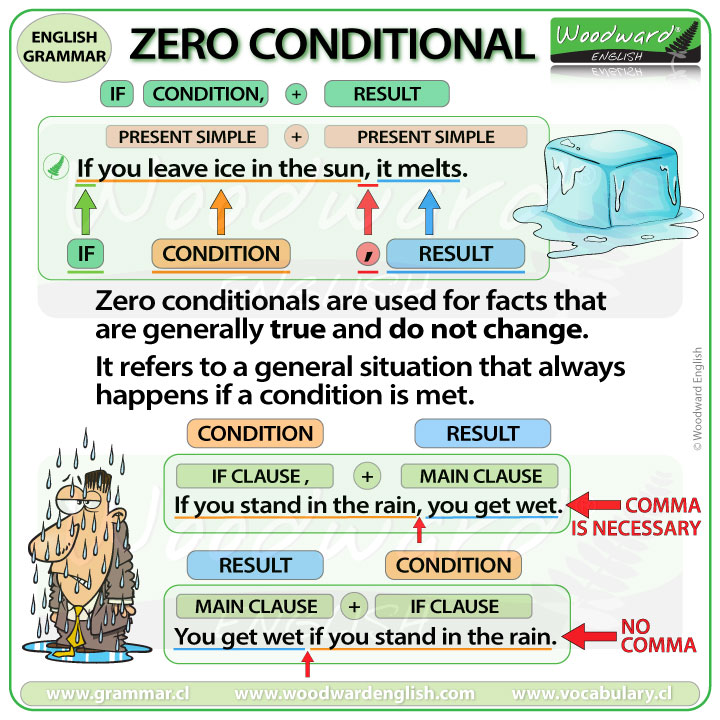Select Page

# Zero Conditional

If you leave ice in the sun, it melts.

This is an example of a zero conditional sentence.
The first part is in the present simple tense and the second part is in the present simple tense too.
What does this mean?

### Zero Conditionals – If clauses

Zero conditionals are used for facts that are generally true and do not change.
It refers to a general situation that always happen (for example in the rules of a game) if a condition is met. The condition always has the same result.

The zero conditional uses the present simple in the if-clause and in the main clause.

Zero Conditionals are also known as Type 0 conditionals (general truth – general rule)

### If + condition, result

Let’s look at this sentence again:

• If you leave ice in the sun, it melts.

The condition is: if you leave ice in the sun.
This is an if-clause because it begins with IF.

What is the result of this if-clause?
The result is: the ice melts. But to avoid repeating the word ICE, we use the pronoun IT so we say: it melts.

Also, we have to use a comma at the end of an if-clause, when an if-clause comes at the beginning of the sentence.

Now, this condition (if you leave ice in the sun) always has the same result (the ice melts). That is why we used the zero conditional with the if-clause, or condition in the present simple tense and the result in the present simple tense, because we are talking about a fact or a general truth.

Let’s look at some other example sentences:

• If you stand in the rain, you get wet.

(The condition is: If you stand in the rain, the result is: you get wet.)
Yes, this is a general truth or fact… if you don’t have an umbrella.

• If you keep milk in the fridge, it lasts longer.

(The condition is: If you keep milk in the fridge, the result is: the milk lasts longer.)
Yes, this is a fact.

• If you put water in the freezer, it becomes ice.

(The condition is: If you put water in the freezer, the result is: the water becomes ice.) This is another fact.

• If I drink coffee at night, it takes me a long time to fall asleep.

(The condition is: If I drink coffee at night, the result is: it takes me a long time to fall asleep.) Yes, this is generally true about me.

All of these sentences begin with an if-clause. Notice how there is comma after the if-clause when this if-clause is at the beginning.

In these examples, what tenses were used?
All of the verbs are in the present simple tense.

So we know we are using the zero conditional, which means all of these sentences refer to a general truth or general fact.

So, we have seen the order of If-clause comma main clause which is the condition + result.

• If you stand in the rain (the condition) you get wet (the result).

A comma is necessary between the two clauses. BUT….

### Result + if + condition

We can also change the order of the sentence and have the main clause (the result) before the if-clause (the condition).

How we can we change our example sentence (If you stand in the rain, you get wet) to this order? We say:

• You get wet if you stand in the rain.

Note that with this order, we do NOT use a comma between the clauses.

Let’s change the order of the example sentences we have already seen.

• Ice melts if you leave it in the sun.
• You get wet if you stand in the rain.
• Milk lasts longer if you keep it in the fridge.
• Water becomes ice if you put it in the freezer.
• It takes me a long time to fall asleep if I drink coffee at night.

### IF / WHEN

We can often use WHEN instead of IF and it has the same meaning.

• If you keep milk in the fridge, it lasts longer.
• When you keep milk in the fridge, it lasts longer.

The result is always the same. One thing (a condition) always leads to another (a result).

Another example:

• If you leave ice in the sun, it melts.
• When you leave ice in the sun, it melts.

There is no difference in meaning when you change IF for WHEN in zero conditional sentences.

### Summary ChartLesson tags: Conditionals, Grammar
Back to: English Course > Conditionals